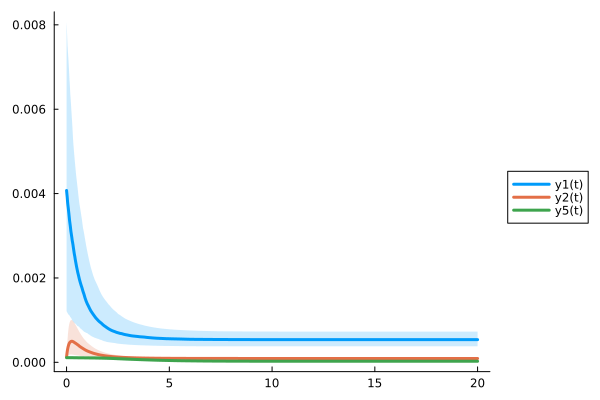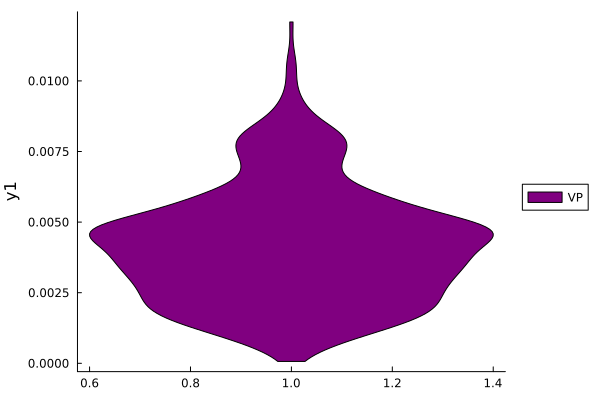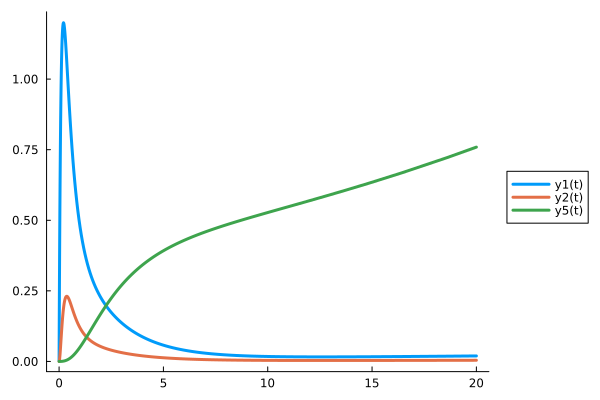# Virtual Population: MCMC

## Julia environment

For this tutorial we will need the following packages:

ModuleDescription
PumasQSPPumasQSP is used to formulate the QSP workflow.
ModelingToolkitThe symbolic modeling environment
DifferentialEquationsThe numerical differential equation solvers
DataSetsWe will load our experimental data from datasets on JuliaHub.
DataFramesHandling tabular data manipulation
TuringBayesian inference with probabilistic programming
Plots and StatsPlotsThe plotting and visualization libraries used for this tutorial.

First we prepare the environment by listing the packages we are using within the example.

# Packages used for this example
using PumasQSP
using ModelingToolkit
using DifferentialEquations
using DataSets
using DataFrames
using CSV
using Turing
using Plots
using StatsPlots

## Model setup

As the example model we are using the Hires model with eight variables. Their dynamics are described by eight equations and default values are set for the variables and parameters. We use the ModelingTookit.jl package to specify the model in Julia.

# Model states and their default values
@variables begin
t
y1(t) = 1.0
y2(t) = 0.0
y3(t) = 0.0
y4(t) = 0.0
y5(t) = 0.0
y6(t) = 0.0
y7(t) = 0.0
y8(t) = 0.0057
end

# Model parameters and their default values
@parameters begin
k1 = 1.71
k2 = 280.0
k3 = 8.32
k4 = 0.69
k5 = 0.43
k6 = 1.81
end

# Differential operator
D = Differential(t)

# Differential equations
eqs = [
D(y1) ~ (-k1*y1 + k5*y2 + k3*y3 + 0.0007),
D(y2) ~ (k1*y1 - 8.75*y2),
D(y3) ~ (-10.03*y3 + k5*y4 + 0.035*y5),
D(y4) ~ (k3*y2 + k1*y3 - 1.12*y4),
D(y5) ~ (-1.745*y5 + k5*y6 + k5*y7),
D(y6) ~ (-k2*y6*y8 + k4*y4 + k1*y5 - k5*y6 + k4*y7),
D(y7) ~ (k2*y6*y8 - k6*y7),
D(y8) ~ (-k2*y6*y8 + k6*y7)
]

# Model definition
@named model = ODESystem(eqs)
\begin{align} \frac{\mathrm{d} \mathrm{y1}\left( t \right)}{\mathrm{d}t} =& 0.0007 + k5 \mathrm{y2}\left( t \right) + k3 \mathrm{y3}\left( t \right) - k1 \mathrm{y1}\left( t \right) \\ \frac{\mathrm{d} \mathrm{y2}\left( t \right)}{\mathrm{d}t} =& k1 \mathrm{y1}\left( t \right) - 8.75 \mathrm{y2}\left( t \right) \\ \frac{\mathrm{d} \mathrm{y3}\left( t \right)}{\mathrm{d}t} =& - 10.03 \mathrm{y3}\left( t \right) + k5 \mathrm{y4}\left( t \right) + 0.035 \mathrm{y5}\left( t \right) \\ \frac{\mathrm{d} \mathrm{y4}\left( t \right)}{\mathrm{d}t} =& - 1.12 \mathrm{y4}\left( t \right) + k3 \mathrm{y2}\left( t \right) + k1 \mathrm{y3}\left( t \right) \\ \frac{\mathrm{d} \mathrm{y5}\left( t \right)}{\mathrm{d}t} =& - 1.745 \mathrm{y5}\left( t \right) + k5 \mathrm{y6}\left( t \right) + k5 \mathrm{y7}\left( t \right) \\ \frac{\mathrm{d} \mathrm{y6}\left( t \right)}{\mathrm{d}t} =& k1 \mathrm{y5}\left( t \right) + k4 \mathrm{y4}\left( t \right) + k4 \mathrm{y7}\left( t \right) - k5 \mathrm{y6}\left( t \right) - k2 \mathrm{y6}\left( t \right) \mathrm{y8}\left( t \right) \\ \frac{\mathrm{d} \mathrm{y7}\left( t \right)}{\mathrm{d}t} =& - k6 \mathrm{y7}\left( t \right) + k2 \mathrm{y6}\left( t \right) \mathrm{y8}\left( t \right) \\ \frac{\mathrm{d} \mathrm{y8}\left( t \right)}{\mathrm{d}t} =& k6 \mathrm{y7}\left( t \right) - k2 \mathrm{y6}\left( t \right) \mathrm{y8}\left( t \right) \end{align}

We are considering two clinical conditions. The first trial (Condition 1) provides a steady state for the initial conditions of the second trial (Condition 2). First we define all parameters of the first trial.

# Read data for Condition 1
data1 = dataset("pumasqsptutorials/hires_trial_1")

params=[k6 => 20.0],
alg=DynamicSS(TRBDF2())
)
SteadyStateTrial with parameters:
k6 => 20.0.


The second condition has altered parameters trial2_params and uses the steady state solution of trial1 as its initial value. Let's also assume that only states y1(t), y2(t) and y5(t) are observed in this condition and set the prior distributions for the respective noise terms for these states.

# Read data for Condition 2
data2 = dataset("pumasqsptutorials/hires_trial_2")

# Clinical condition 2
trial2_params = [k3 => 4.0, k4 => 0.4, k6 => 8.0]
trial2_tspan = (0.0, 20.0)

trial2_save_names = [y1, y2, y5]

trial2_noise_priors = [y1 => InverseGamma(2,2),
y2 => InverseGamma(2,3),
y5 => InverseGamma(2,1)]
3-element Vector{Pair{Symbolics.Num, Distributions.InverseGamma{Float64}}}:
y1(t) => Distributions.InverseGamma{Float64}(
invd: Distributions.Gamma{Float64}(α=2.0, θ=0.5)
θ: 2.0
)

y2(t) => Distributions.InverseGamma{Float64}(
invd: Distributions.Gamma{Float64}(α=2.0, θ=0.3333333333333333)
θ: 3.0
)

y5(t) => Distributions.InverseGamma{Float64}(
invd: Distributions.Gamma{Float64}(α=2.0, θ=1.0)
θ: 1.0
)


We are now ready to define trial2. By default likelihood in this case is a multivariate Normal distribution with a vector of mean values equal to the saved states ([y1(t), y2(t), y5(t)]) at each timepoint and a vector of standard deviations, derived from the respective priors (trial2_noise_priors).

# Create the Trial object for trial 2
trial2 = Trial(data2, model;
forward_u0 = true,
params = trial2_params,
tspan = trial2_tspan,
save_names = trial2_save_names,
noise_priors = trial2_noise_priors,
alg = Rosenbrock23(),
abstol=1e-9, reltol=1e-8
)
Trial with parameters:
k3 => 4.0
k4 => 0.4
k6 => 8.0
and initial conditions obtained from another trial.
timespan: (0.0, 20.0)


## Analysis

Next we define the InverseProblem, which, for MCMC methods, holds the prior distributions of all parameters and initial conditions to be optimized, as its search space.

# Define a search space
example_ss = [k1 => TransformedBeta(Beta=Beta(2,6), lb=1.0, ub=3.0),
k2 => TransformedBeta(Beta=Beta(2,2), lb=200.0, ub=400.0),
y1 => TransformedBeta(Beta=Beta(2,5), lb=0.0, ub=3.0)
]

# Create sequence of Steady State Trials

# Create an InverseProblem object
prob = InverseProblem(trials, model, example_ss)
InverseProblem for model with 2 trials optimizing 3 parameters and states.


Once the QSP model and all trials are defined, the package can generate potential patients, i.e. find parameter combinations for the provided model, which describe the trial data sufficiently well. Particularly in the case of MCMC methods, the posterior distribution of the unknown parameters given the data will be approximated in a sampling-based manner.

# Generate potential population
vp = vpop(prob,
MCMCOpt(maxiters = 1000, sampler = NUTS(0.65), discard_initial = 500);
population_size = 500)
MCMC result of length 500 computed in 2 minutes and 9 seconds.
┌────────────┬─────────┬─────────┐
│      y1(t) │      k1 │      k2 │
├────────────┼─────────┼─────────┤
│  0.0057917 │ 1.28888 │ 296.801 │
│ 0.00264983 │ 1.58909 │ 268.097 │
│ 0.00224626 │ 1.22059 │  313.01 │
│ 0.00439438 │ 1.27885 │ 283.965 │
│ 0.00370099 │ 1.40809 │ 348.382 │
│ 0.00587055 │ 1.74316 │ 309.192 │
│ 0.00826058 │ 1.92291 │ 222.915 │
│ 0.00315886 │ 1.68269 │ 291.937 │
│ 0.00139706 │ 1.66325 │  353.79 │
│ 0.00966692 │ 1.47832 │ 373.281 │
│ 0.00538329 │ 1.39459 │ 337.151 │
│  0.0030097 │ 2.14366 │ 317.561 │
│ 0.00479104 │ 1.09172 │  245.31 │
│ 0.00535953 │   1.101 │ 309.826 │
│ 0.00542087 │  1.4192 │ 329.763 │
│ 0.00460814 │ 2.02418 │ 319.681 │
│     ⋮      │    ⋮    │    ⋮    │
└────────────┴─────────┴─────────┘
484 rows omitted


Finally, the original MCMCChain object that was created during Bayesian inference can be accessed via:

vp.original
Chains MCMC chain (1000×19×1 Array{Float64, 3}):

Iterations        = 501:1:1500
Number of chains  = 1
Samples per chain = 1000
Wall duration     = 112.79 seconds
Compute duration  = 112.79 seconds
parameters        = y1(t), k1, k2, noise, noise[2, y1(t)], noise[2, y2(t)], noise[2, y5(t)]
internals         = lp, n_steps, is_accept, acceptance_rate, log_density, hamiltonian_energy, hamiltonian_energy_error, max_hamiltonian_energy_error, tree_depth, numerical_error, step_size, nom_step_size

Summary Statistics
parameters       mean       std      mcse    ess_bulk   ess_tail      r ⋯
Symbol    Float64   Float64   Float64     Float64    Float64   Floa ⋯

y1(t)     0.0041    0.0021    0.0001    727.4417   613.4829    1.0 ⋯
k1     1.5250    0.2944    0.0090   1043.3271   591.5385    0.9 ⋯
k2   301.8013   43.1041    1.4642    844.7945   699.6500    0.9 ⋯
noise     0.5947    0.2627    0.0088   1071.4067   526.4931    1.0 ⋯
noise[2, y1(t)]     0.0161    0.0015    0.0001    594.3668   766.5140    0.9 ⋯
noise[2, y2(t)]     0.0240    0.0021    0.0001    957.2142   687.7433    1.0 ⋯
noise[2, y5(t)]     0.0080    0.0007    0.0000    954.5615   632.8908    1.0 ⋯
2 columns omitted

Quantiles
parameters       2.5%      25.0%      50.0%      75.0%      97.5%
Symbol    Float64    Float64    Float64    Float64    Float64

y1(t)     0.0007     0.0025     0.0040     0.0054     0.0087
k1     1.0872     1.2939     1.4944     1.6995     2.1825
k2   222.0308   270.4760   301.8101   334.5487   378.4413
noise     0.2722     0.4170     0.5345     0.7106     1.2671
noise[2, y1(t)]     0.0135     0.0151     0.0160     0.0171     0.0190
noise[2, y2(t)]     0.0202     0.0226     0.0239     0.0254     0.0283
noise[2, y5(t)]     0.0067     0.0075     0.0079     0.0084     0.0095


The first index of the noise parameters is the trial index and the second one is the state that it corresponds to. In the first trial, it was assumed that the same noise parameter is applied to all states, so there is only one index in noise.

## Visualization

We can conveniently analyze and visualize the results by relying on the ploting recipes for Pumas-QSP.

# Viusalization (1/2): Simulation results for Condition 2
plot(vp, trials, legend = :outerright, grid = "off")# Viusalization (2/2): Parameters
params = DataFrame(vp)[!,"y1(t)"]
plt = violin(params, grid = :off, legend = :outerright, label = "VP", ylabel = "y1", color = "purple")## Simulating Virtual Trials

Once we have created and validated our VP, we can use it to explore the system for new clinical conditions. For instance, what would happen to trial2 if the initial condition for y3 and the parameter k1 were different? For this, we can use the virtual_trial function.

# Create virtual trial
vt = virtual_trial(trials, model;
u0 = [y3 =>2.1],
params = [k1 => 1.9],
tspan = (0, 100))

# Visualize simulation results
plot(vp, vt, legend = :outerright, grid = "off")